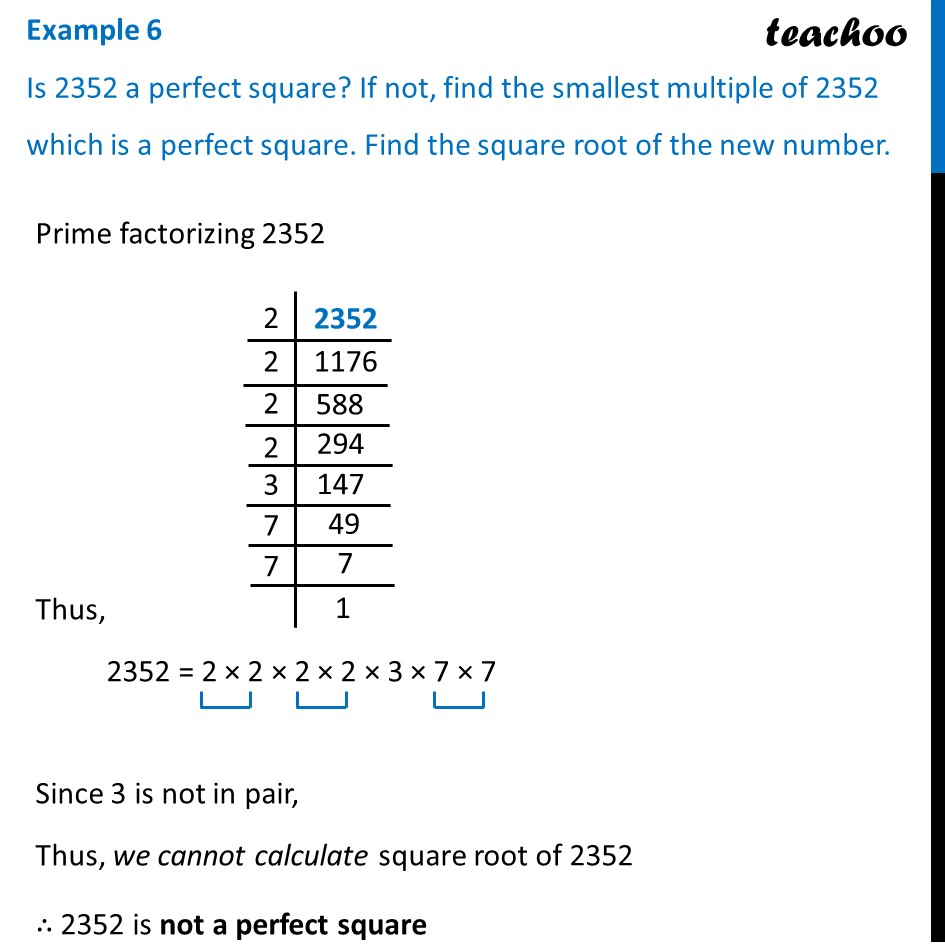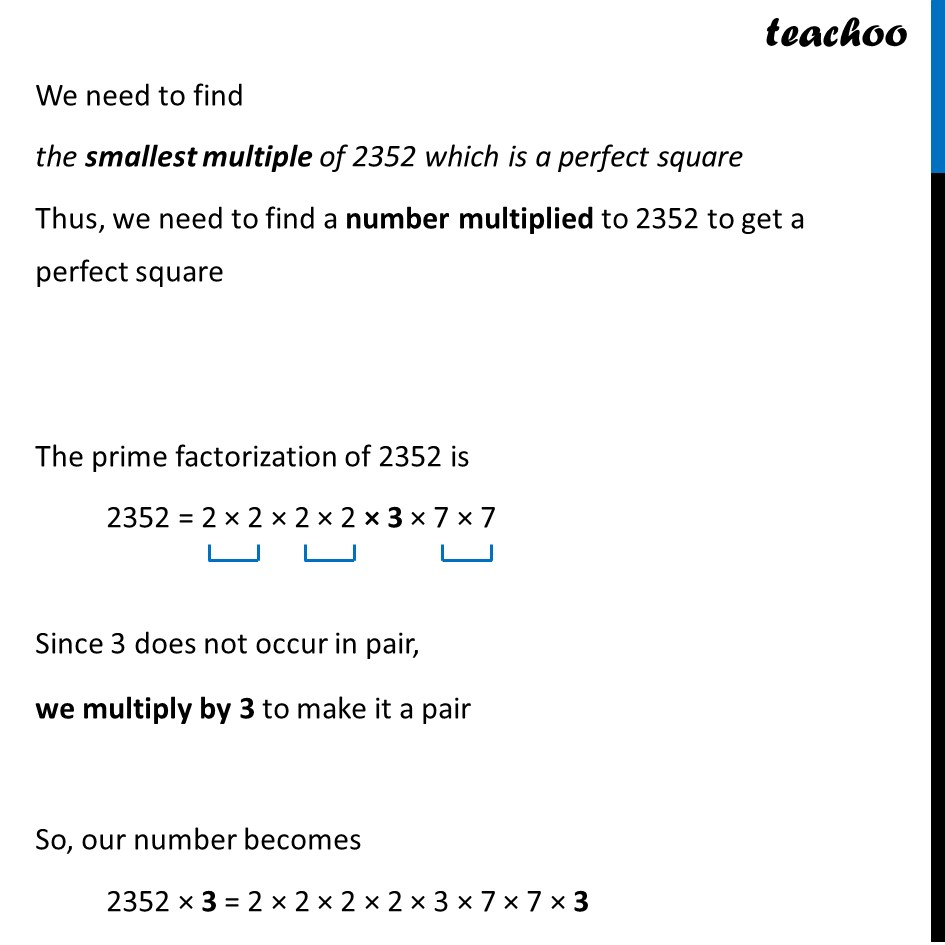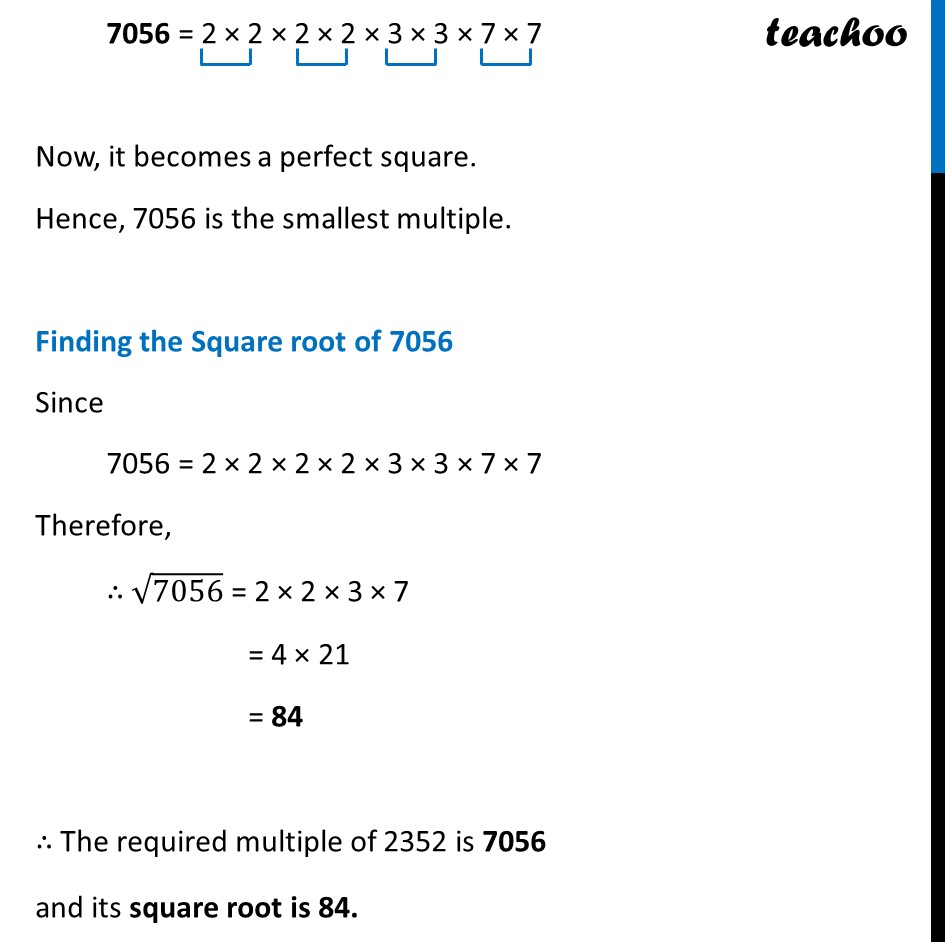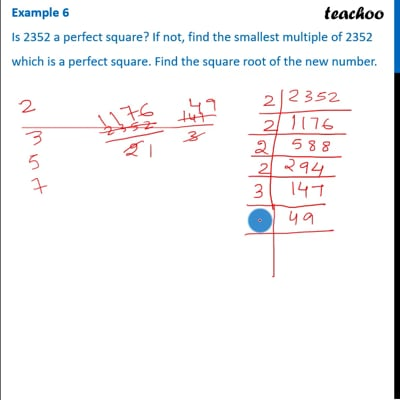Examples

Chapter 6 Class 8 Squares and Square Roots
Serial order wiseThis video is only available for Teachoo black users

Introducing your new favourite teacher - Teachoo Black, at only ₹83 per month

### Transcript

Example 6 Is 2352 a perfect square? If not, find the smallest multiple of 2352 which is a perfect square. Find the square root of the new number.Prime factorizing 2352 Prime factorizing 2352 Thus, 2352 = 2 × 2 × 2 × 2 × 3 × 7 × 7 Since 3 is not in pair, Thus, we cannot calculate square root of 2352 ∴ 2352 is not a perfect square We need to find the smallest multiple of 2352 which is a perfect square Thus, we need to find a number multiplied to 2352 to get a perfect square The prime factorization of 2352 is 2352 = 2 × 2 × 2 × 2 × 3 × 7 × 7 Since 3 does not occur in pair, we multiply by 3 to make it a pair So, our number becomes 2352 × 3 = 2 × 2 × 2 × 2 × 3 × 7 × 7 × 3 7056 = 2 × 2 × 2 × 2 × 3 × 3 × 7 × 7 Now, it becomes a perfect square. Hence, 7056 is the smallest multiple. Finding the Square root of 7056 Since 7056 = 2 × 2 × 2 × 2 × 3 × 3 × 7 × 7 Therefore, ∴ √7056 = 2 × 2 × 3 × 7 = 4 × 21 = 84 ∴ The required multiple of 2352 is 7056 and its square root is 84.